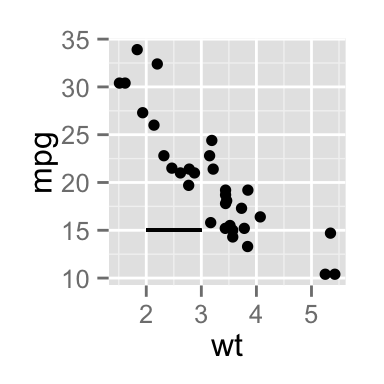# ggplot2 add straight lines to a plot : horizontal, vertical and regression lines

This tutorial describes how to add one or more straight lines to a graph generated using R software and ggplot2 package.

The R functions below can be used :

• geom_hline() for horizontal lines
• geom_abline() for regression lines
• geom_vline() for vertical lines

# geom_hline : Add horizontal lines

A simplified format of the function geom_hline() is :

``geom_hline(yintercept, linetype, color, size)``

It draws a horizontal line on the current plot at the specified ‘y’ coordinates :

``````library(ggplot2)
# Simple scatter plot
sp <- ggplot(data=mtcars, aes(x=wt, y=mpg)) + geom_point()
# Add horizontal line at y = 2O
sp + geom_hline(yintercept=20)
# Change line type and color
sp + geom_hline(yintercept=20, linetype="dashed", color = "red")
# Change line size
sp + geom_hline(yintercept=20, linetype="dashed",
color = "red", size=2)``````Read more on line types here : Line types in R

# geom_vline : Add vertical lines

A simplified format of the function geom_vline() is :

``geom_vline(xintercept, linetype, color, size)``

It draws a vertical line on the current plot at the specified ‘x’ coordinates :

``````library(ggplot2)
# Add a vertical line at x = 3
sp + geom_vline(xintercept = 3)
# Change line type, color and size
sp + geom_vline(xintercept = 3, linetype="dotted",
color = "blue", size=1.5)``````# geom_abline : Add regression lines

A simplified format of the function geom_abline() is :

``geom_abline(intercept, slope, linetype, color, size)``

The function lm() is used to fit linear models.

``````# Fit regression line
require(stats)
reg<-lm(mpg ~ wt, data = mtcars)
reg``````
``````##
## Call:
## lm(formula = mpg ~ wt, data = mtcars)
##
## Coefficients:
## (Intercept)           wt
##      37.285       -5.344``````
``````coeff=coefficients(reg)
# Equation of the line :
eq = paste0("y = ", round(coeff,1), "*x + ", round(coeff,1))
# Plot
sp + geom_abline(intercept = 37, slope = -5)+
ggtitle(eq)
# Change line type, color and size
sp + geom_abline(intercept = 37, slope = -5, color="red",
linetype="dashed", size=1.5)+
ggtitle(eq)``````Note that, the function stat_smooth() can be used for fitting smooth models to data.

``sp + stat_smooth(method="lm", se=FALSE)``# geom_segment : Add a line segment

A simplified format of the function geom_segment() is :

``geom_segment(aes(x, y, xend, yend))``

It’s possible to use it as follow :

``````# Add a vertical line segment
sp + geom_segment(aes(x = 4, y = 15, xend = 4, yend = 27))
sp + geom_segment(aes(x = 2, y = 15, xend = 3, yend = 15))``````Note that, you can add an arrow at the end of the segment. grid package is required

``````library(grid)
sp + geom_segment(aes(x = 5, y = 30, xend = 3.5, yend = 25),
arrow = arrow(length = unit(0.5, "cm")))``````# Infos

This analysis has been performed using R software (ver. 3.1.2) and ggplot2 (ver. )